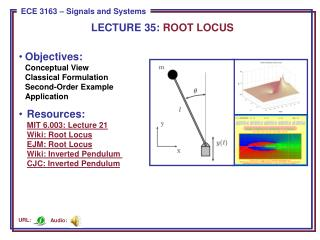# Objectives: Conceptual View Classical Formulation Second-Order Example Application - PowerPoint PPT PresentationDownload PresentationObjectives: Conceptual View Classical Formulation Second-Order Example Application

Objectives: Conceptual View Classical Formulation Second-Order Example ApplicationDownload Presentation## Objectives: Conceptual View Classical Formulation Second-Order Example Application

- - - - - - - - - - - - - - - - - - - - - - - - - - - E N D - - - - - - - - - - - - - - - - - - - - - - - - - - -
##### Presentation Transcript

1. LECTURE 35: ROOT LOCUS • Objectives: • Conceptual ViewClassical FormulationSecond-Order ExampleApplication • Resources:MIT 6.003: Lecture 21Wiki: Root LocusEJM: Root LocusWiki: Inverted Pendulum CJC: Inverted Pendulum Audio: URL:

2. The Concept of a Root Locus • Recall our simple control systemwith transfer function: • The controllers C(s) and G(s) can bedesigned to stabilize the system, but that could involve a multidimensional optimization. Instead, we would like a simpler, more intuitive approach to understand the behavior of this system. • Recall the stability of the system depends on the poles of 1 + C(s)G(s)P(s). • A root locus, in its most general form, is simply a plot of how the poles of our transfer function vary as the parameters of C(s) and G(s) are varied. • The classic root locus problem involves a simplified system: Closed-loop poles are the same.

3. Example: First-Order System • Consider a simple first-order system: • The pole is at s0 = -(2+K). Vary Kfrom 0 to : • Observation: improper adjustment of the gain can cause the overall system to become unstable. Becomes less stable Becomes more stable

4. Example: Second-Order System With Proportional Control • Using Black’s Formula: • How does the step responsevary as a function of the gain, K? • Note that as K increases, thesystem goes from too little gainto too much gain.

5. How Do The Poles Move? Desired Response • Can we generalize this analysis to systems of arbitrary complexity? • Fortunately, MATLAB has support for generation of the root locus: • num = ; • den = [1 101 101]; (assuming K = 1) • P = tf(num, den); • rlocus(P);

6. More General Case • Assume no pole/zero cancellation in G(s)H(s): • Closed-loop poles are the roots of: • It is much easier to plot the root locus for high-order polynomials because we can usually determine critical points of the plot from limiting cases(e.g., K= 0, ), and then connect the critical points using some simple rules. • The root locus is defined as traces of s for unity gain: • Some general rules: • At K= 0, G(s0)H(s0) =   s0are the poles of G(s)H(s). • At K= , G(s0)H(s0) = 0 s0are the zeroes of G(s)H(s). • Rule #1: start at a pole at K= 0 and end at a zero at K= . • Rule #2: (K  0) number of zeroes and poles to the right of the locus point must be odd.

7. Example

8. Inverted Pendulum • Pendulum which has its mass above its pivot point. • It is often implemented with the pivot point mounted on a cart that can move horizontally. • A normal pendulum is stable when hanging downwards, an inverted pendulum is inherently unstable. • Must be actively balanced in order to remain upright, either by applying a torque at the pivot point or by moving the pivot point horizontally (Wiki).

9. Feedback System – Use Proportional Derivative Control • Equations describing the physics: • The poles of the system are inherentlyunstable. • Feedback control can be used to stabilize both the angle and position. • Other approaches involve oscillatingthe support up and down.

10. Feedback System – Implementation

11. Summary • Reviewed how stability is a function of gain for a simple feedback control circuit. • Introduced the root locus diagram as an analysis tool to understand stability. • Demonstrated its calculation for some simple first and second-order systems. • Discussed an application of feedback control involving stabilization of an inverted pendulum.